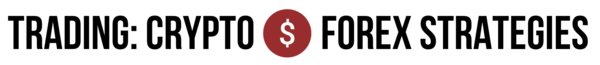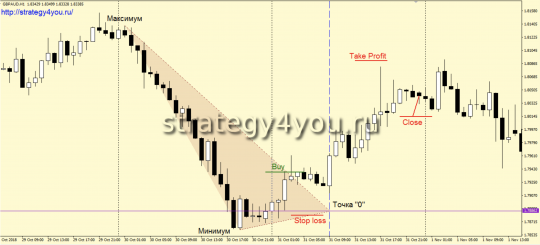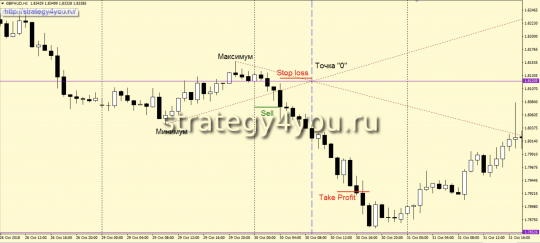# +273% for 12 months on GBP/AUD (H1) – Forex Strategy “Magic Triangle”

Forex strategy «Magic Triangle» is a rather unusual and even strange trading system. Despite the rather controversial way of trading, we still decided to introduce you to this trading method. To apply this strategy, a trader does not need any special knowledge and analysis skills, it is enough just to be able to use the trading terminal.

• Only trading time can be attributed to the system’s disadvantages: the signal is expected from 00:00 to 10:00 of THIS Broker’s terminal time (EST).

Statistics:

From April 2018 to April 2019, the trading system showed a positive result in the region of +273% with a risk per trade of 3%. The maximum drawdown is 15%.

• Time interval — H1.
• Currency pair — GBP/AUD.
• Indicators are not required.1) At the opening of a new trading day, you should determine the high and low of the closed day.

2) We also draw a horizontal line at the opening price of a new day.

3) Draw a vertical line according to the level of the future 9-hour candle.

4) The point of intersection of these two lines will be called point «0».

5) The maximum and minimum of the previous trading day should be connected by lines with the point «0».

6) Thus, a kind of triangle is formed.

7) If the next candle closes above the upper line of this triangle (it is the closing price that is of interest), then a buy deal is concluded at the opening of the next candle.

8) Stop loss is set 5 points below the «0» point. The minimum stop size is 30 and the maximum stop is 60 pips.

9) After passing 50 points in the positive zone, the transaction is transferred to breakeven.

10) Take profit is three times the stop loss order.

11) If the trade is still active at the close of the day, then it should be closed at the market price.

12) If the triangle is built in such a way that both of its lines are ascending or descending, then we do not trade on this day.

13) If the first closing price outside the triangle zone is located at a distance of more than 40 points from the triangle, then we also do not trade on this day.

## Conditions for transactions for sale:1) At the opening of a new trading day, you should determine the MAX and MIN of the already closed day.

2) Draw a horizontal line at the opening price of a new day (purple in the figure above).

3) According to the level of the future 9-hour candle, we draw a vertical line (in the figure — a blue dotted line).

4) The point where these 2 lines intersect will be called the «0» point.

5) MAX and MIN of the previous day should be connected by lines with «0».

6) As a result, we get a kind of triangle.

7) If the next H1 candlestick closes below the lower line of the resulting triangle (it is the closing price that is of interest), then a sell trade is opened at the opening of the next H1 candlestick.

8) A safety stop loss must be set 5 points above the “0” point. The minimum stop size is 30 pips and the maximum is 60.

9) After the price passes 50 points in the «+» zone, the transaction is transferred to the breakeven point.

10) Profit taking order — take profit is 3 times more than the set stop loss.

11) If at the close of the day the deal is still not closed, then it must still be closed at the current market price.

12) If the resulting triangle is built in such a way that 2 of its lines are ascending or descending, then there is no need to trade on this day.

13) If the 1st closing price of the candle outside the triangle zone is located at a distance of more than 40 points from its lines, then there is no need to trade on this day either.

## Video version of the Magic Triangle forex strategy:

• Recommended to watch with English subtitles!

• There are no indicators, so there is no template for MT4 either.
• Strategy test for 12 months: +286.76%, see more here >>

Original